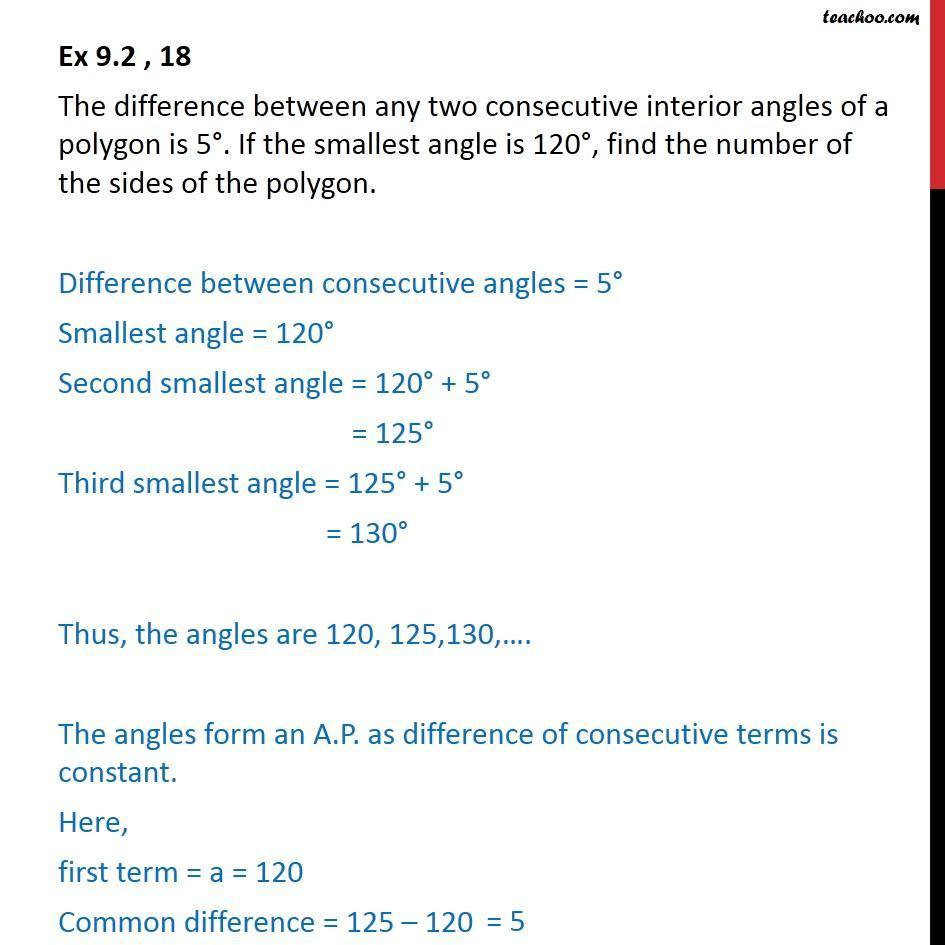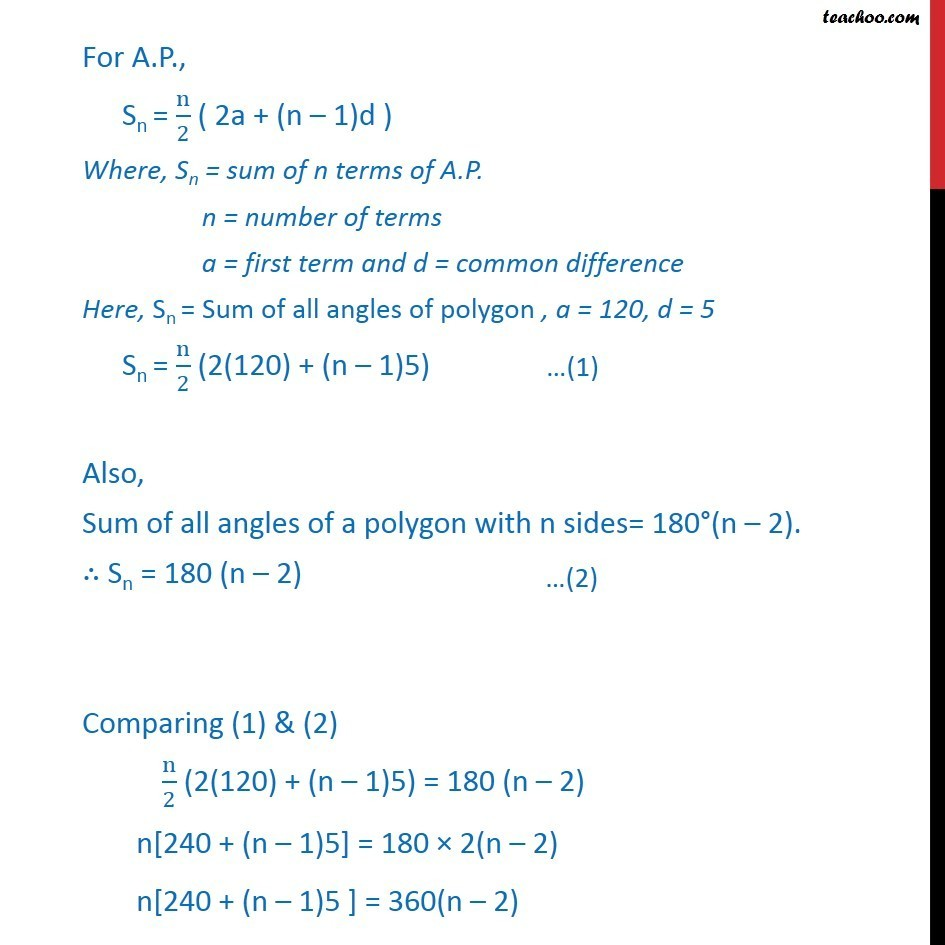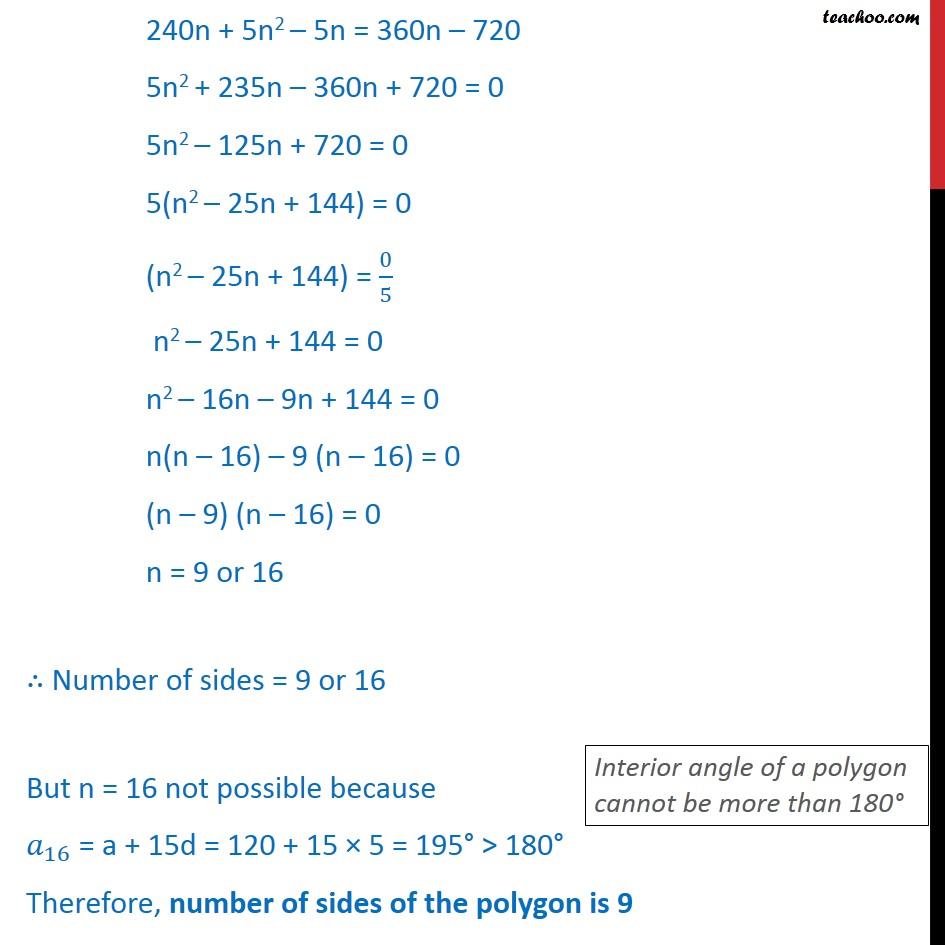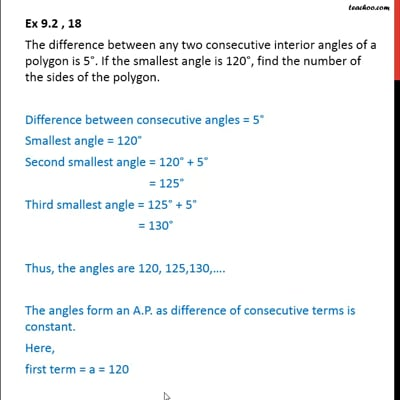Ex 9.2

Chapter 9 Class 11 Sequences and Series (Term 1)
Serial order wiseThis video is only available for Teachoo black users

### Transcript

Ex 9.2 , 18 The difference between any two consecutive interior angles of a polygon is 5 . If the smallest angle is 120 , find the number of the sides of the polygon. Difference between consecutive angles = 5 Smallest angle = 120 Second smallest angle = 120 + 5 = 125 Third smallest angle = 125 + 5 = 130 Thus, the angles are 120, 125,130, . The angles form an A.P. as difference of consecutive terms is constant. Here, first term = a = 120 Common difference = 125 120 For A.P., Sn = n/2 ( 2a + (n 1)d ) Where, Sn = sum of n terms of A.P. n = number of terms a = first term and d = common difference Here, Sn = Sum of all angles of polygon , a = 120, d = 5 Sn = n/2 (2(120) + (n 1)5) Also, Sum of all angles of a polygon with n sides= 180 (n 2). Sn = 180 (n 2) Comparing (1) & (2) n/2 (2(120) + (n 1)5) = 180 (n 2) n[240 + (n 1)5] = 180 2(n 2) n[240 + (n 1)5 ] = 360(n 2) 240n + 5n2 5n = 360n 720 5n2 + 235n 360n + 720 = 0 5n2 125n + 720 = 0 5(n2 25n + 144) = 0 (n2 25n + 144) = 0/5 n2 25n + 144 = 0 n2 16n 9n + 144 = 0 n(n 16) 9 (n 16) = 0 (n 9) (n 16) = 0 n = 9 or 16 Number of sides = 9 or 16